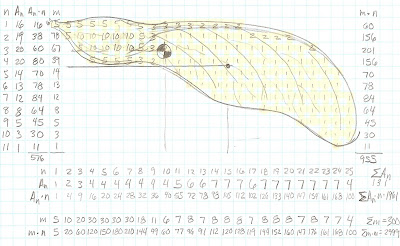## Tuesday, April 20, 2010

### Maple Seed DynamicsI am starting on the Maple Seed Helicopter problem.

I want to begin by studying the mass and geometric properties of the seed. After something is known about these properties I will set out to study the dynamic motion.

In this figure I have sketched out a maple seed and broken it into discrete "squares". Each of the squares is assigned a relative mass number such as 1, 3, 5 and 10. The squares also represent discrete areas of the geometry (DeltaX by DeltaY if you will). The discrete columns and rows are identified by "n". A-n represents the area of any given strip in the subject column or row. These areas A-n are multiplied by the values of "n" which also represents the distance of the respective areas from a set zero datum. Summations are made of the area moments (A-n*n) and the mass moments (m*n). Approximate mass and area centroids are calculated and marked on the sketch. The black/white target marks the mass centroid and the dot down and to the right marks the geometric centroid.

We know that the seed will spin about some axis passing through the mass centroid. The angle of the axis, the rotational speed, pitch, falling velocity, etc.. will be part of the solution to be sought.

The geometric centroid seems to be an important quantity. I feel that the air resistance acting on the plan view area will be centered at this geometric centroid. The force will create a moment causing the seed to pitch downward. This will be part of the investigation to be completed in the coming days.

Irasohn.